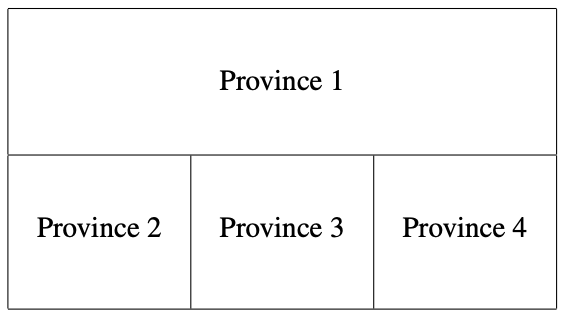시간 제한메모리 제한제출정답맞힌 사람정답 비율
1 초 512 MB222100.000%

## 문제

A national election will be held in the JOI kingdom. The JOI kingdom consists of N provinces.

The map of the JOI kingdom is a rectangular-shaped grid consisting of H ×W blocks. There are H blocks in the vertical direction, and there are W blocks in the horizontal direction. A block is connected with another block by one of the four sides (left, right, top, bottom) of it. The H × W blocks are divided into N provinces. Each province is a connected region consisting of blocks. In total, there are Pi voters in the i-th province (1 ≤ i ≤ N).

You are the chairman of the National Election Committee of JOI. Your task is to divide the N provinces into K electoral districts (1 ≤ K ≤ N) in order to elect K representatives. Each electoral district should contain at least one province, and the blocks belonging to an electoral district should form a connected region. Note that a block is connected with another block by one of the four sides (left, right, top, bottom). Two blocks sharing only a vertex are not connected.

For each electoral district, the ratio 1/(the number of voters in the electoral district) is called the weight of a single vote of the electoral district. The vote-value disparity is defined to be the maximal weight of a single vote for all electoral districts divided by the the minimal weight of a single vote for all electoral districts.

Recently, the value of “the vote-value disparity” becomes a serious social issue. You have to make this value as small as possible.

Given the information of the provinces of the JOI kingdom and the number of representatives, determine how to divide the provinces into electoral districts so that the value of the vote-value disparity is as small as possible.

## 입력

Read the following data from the standard input.

• The first line of input contains four space separated integers H, W, N, K, where the height of the map of the JOI kingdom is H, the width is W, the number of provinces is N, the number of representatives is K.
• Each of the following H lines contains W space separated integers. In the i-th line (1 ≤ i ≤ H), the j-th integer (j ≤ j ≤ W) is Sij (1 ≤ Sij ≤ N). This means the block in i-th row from above and the j-th column from left belongs to the Sij-th province.
• In the i-th line (1 ≤ i ≤ N) of the following N lines contains an integer Pi, the number of voters in the i-th province.

## 출력

Write the way to divide the provinces into electoral districts in N lines. The i-th line (1 ≤ i ≤ N) of output should contain an integer, the number of the electoral district where the i-th province belongs.

## 제한

• 1 ≤ H ≤ 200
• 1 ≤ W ≤ 200
• 1 ≤ N ≤ 10 000
• 1 ≤ K ≤ N
• 1 ≤ Pi ≤ 100 000 (1 ≤ i ≤ N)
• For each province, the blocks belonging to the province form a connected region.

## 점수

For each input, your score is calculated as follows.

If your output does not satisfy the condition in the task statement, your score is 0. If it satisfies the condition, let D denote the vote-value disparity of your output. Then your score is calculated by the following way.

• If Y < D, your score is 0.
• If X < D ≤ Y, your score is the value of ((Y − D)/(Y − X))2 × 100 truncated to zero decimal places.
• If D ≤ X, your score is 100.

The values of X, Y are given in the following table.

Input X Y
01.txt 1.02 2
02.txt 1.66 2.5
03.txt 1.06 2.5
04.txt 1.005 3
05.txt 1.0005 2

## 예제 입력 1

2 3 4 3
1 1 1
2 3 4
3
5
7
10


## 예제 출력 1

1
2
1
3


## 힌트

In this example, the shape of the JOI kingdom is as follows.The number of voters in each province is 3, 5, 7, 10, respectively. In this sample output, the provinces are divided into electoral districts as follows.

• Electoral District 1 : Province 1 and Province 3
• Electoral District 2 : Province 2
• Electoral District 3 : Province 4

The number of voters in each electoral district is 10, 5, 10, respectively. The weight of a single vote for each electoral district is 0.1, 0.2, 0.1, respectively. Therefore, the vote-value disparity is 0.2/0.1 = 2. If X = 1.5, Y = 3, we have ((3−2)/(3−1.5))2 × 100 = 44.4444444444··· and this output is worth 44.4444444444··· points.

In this sample input, the following division is not allowed because the Electoral District 2 does not form a connected region.

• Electoral District 1 : Province 1
• Electoral District 2 : Province 2 and Province 4
• Electoral District 3 : Province 3

Text

## 채점 및 기타 정보

• 100점 이상을 획득해야 를 받는다.
• 예제는 채점하지 않는다.